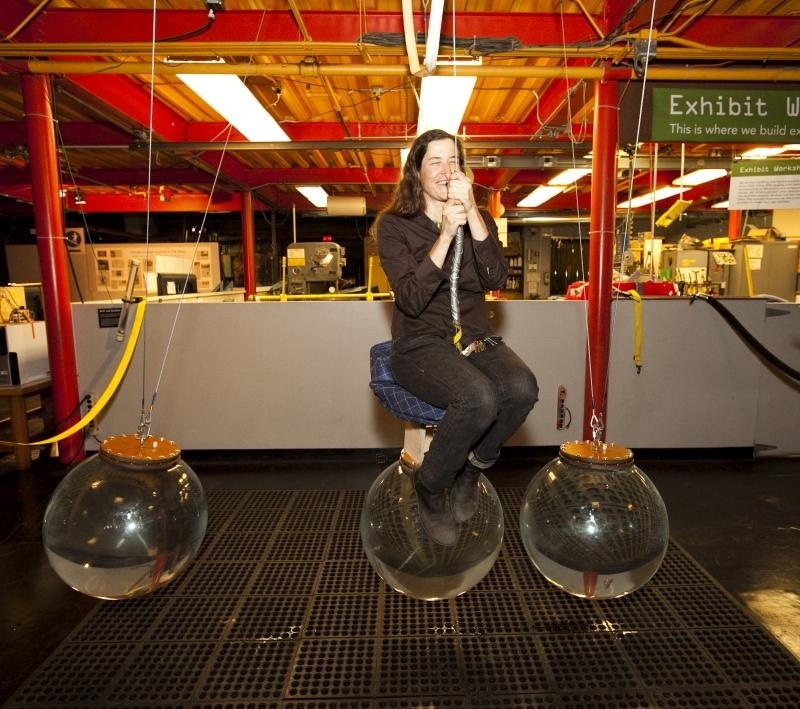You need to conserve MV and MV^2A rideable Newton's Cradle at the Exploratorium.

Introduction

Isaac Newton and his contemporaries studied collisions of balls hanging from pendulums. When two identical balls collided after one was drawn aside and released the moving ball came to rest and the other ball moved on with the same velocity the moving ball had just before the collision. To understand this collision we needed to use both conservation of momentum and energy.

Material

A newton's cradle. (Some small ones are commercially available for under \$5.)(If you build one leave a small gap between the balls)

To Do and Notice

Pull one ball to the side and release it.

Notice that the ball comes to rest when it hits the other balls and one ball departs the far end and rises to the same height from which the first ball was released.

What's Going On?

Conservation of momentum alone works when applied to the above experiment. All balls have the same mass M, which we can assume to be equal to 1.0 unit of mass, for example 1 kg

We can also assume that the incoming ball has a velocity V = 1.0 unit. For example 1 m/s

Conservation of momentum means Mi Vi = Mf Vf  the final momentum equals the initial momentum.

In the case were one ball move off with the same velocity as the initial ball we find  1* 1 = 1*1  = 1,so momentum is conserved.

What prevents 2 balls from moving off with 1/2 the initial velocity where we would have 1*1 = 1*0.5 + 1* 0.5 and momentum is conserved.

Let's look at energy 0.5 MiVi^2 = 0.5 MfVf^2, cancel the 1/2s and check the result for one ball coming out with the same initial velocity.

1*1^2 = 1 * 1^2  and energy is conserved.

now let's look at the case where 2 balls come out with 1/2 the energy.

1 * 1^2 = 1 * (1/2)^2 + 1 * (1/2)^2 = 1/2  and since 1 does not equal 1/2 energy is not conserved so by applying both conservation laws we can understand why just one ball carries away the motion of the incoming ball.

Going Further

You can go on by pulling aside two balls. Notice that two balls bounce cot from the far end of the ball chain.

As long as there is a slight gap between all the balls in the chain you can treat the two ball collision as two one ball collisions.

The first ball of the two hits and knocks one ball out of the far end, then the second ball hits and knocks the second ball out.

This is one of the great tools of mathematics, where a problem is reduced to one you have already solved.

Etc

When one ball strikes another the energy of the collision impact on one side of the ball moves through the ball at the speed of sound in the ball which is much faster than the speed of the ball itself.

Wikipedia has a great article on the Newton's Cradle.